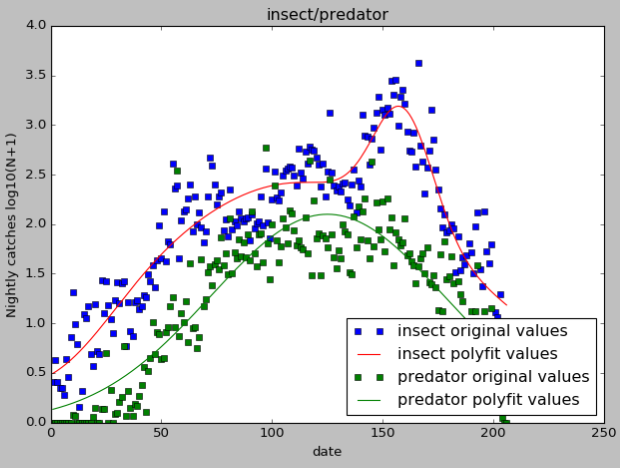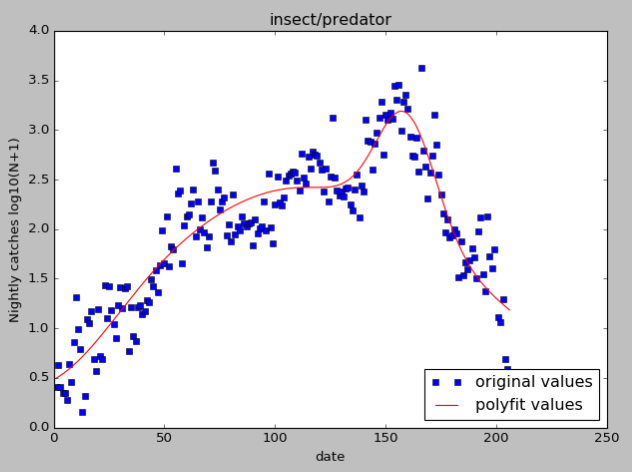• python散点图拟合曲线
千次阅读
2020-11-01 12:49:45

http://blog.sina.com.cn/s/blog_aed5bd1d0102vid7.html

1.多项式拟合范例：

import matplotlib.pyplot as plt

import numpy as np

#潘海东,2014/1/13

x = np.arange(1, 17, 1)

y = np.array([4.00, 6.40, 8.00, 8.80, 9.22, 9.50, 9.70, 9.86, 10.00, 10.20, 10.32, 10.42, 10.50, 10.55, 10.58, 10.60])

z1 = np.polyfit(x, y, 3)#用3次多项式拟合

p1 = np.poly1d(z1)

print(p1) #在屏幕上打印拟合多项式

yvals=p1(x)#也可以使用yvals=np.polyval(z1,x)

plot1=plt.plot(x, y, '*',label='original values')

plot2=plt.plot(x, yvals, 'r',label='polyfit values')

plt.xlabel('x axis')

plt.ylabel('y axis')

plt.legend(loc=4)#指定legend的位置,读者可以自己help它的用法

plt.title('polyfitting')

plt.show()

plt.savefig('p1.png')2.指定函数拟合

#潘海东,2014/1/13

#使用非线性最小二乘法拟合

import matplotlib.pyplot as plt

from scipy.optimize import curve_fit

import numpy as np

#用指数形式来拟合

x = np.arange(1, 17, 1)

y = np.array([4.00, 6.40, 8.00, 8.80, 9.22, 9.50, 9.70, 9.86, 10.00, 10.20, 10.32, 10.42, 10.50, 10.55, 10.58, 10.60])

def func(x,a,b):

return a*np.exp(b/x)

popt, pcov = curve_fit(func, x, y)

a=popt#popt里面是拟合系数，读者可以自己help其用法

b=popt

yvals=func(x,a,b)

plot1=plt.plot(x, y, '*',label='original values')

plot2=plt.plot(x, yvals, 'r',label='curve_fit values')

plt.xlabel('x axis')

plt.ylabel('y axis')

plt.legend(loc=4)#指定legend的位置,读者可以自己help它的用法

plt.title('curve_fit')

plt.show()

plt.savefig('p2.png')更多相关内容
• 广告关闭腾讯云11.11云上盛惠 ，精选热门产品助力... 代码创建了x和y之间的散点图，我需要一段代码来过度绘制一条最适合散点图中的数据的行，而且我没有一个内置的pylab函数对我有用。 from matplotlib import *from...广告关闭

腾讯云11.11云上盛惠 ，精选热门产品助力上云，云服务器首年88元起，买的越多返的越多，最高返5000元！

下面是我在文本文件中绘制数据散点图的代码。 我打开的文件包含两列。 左列为x坐标，右列为y坐标。 代码创建了x和y之间的散点图，我需要一段代码来过度绘制一条最适合散点图中的数据的行，而且我没有一个内置的pylab函数对我有用。 from matplotlib import *from pylab import * with open(file.txt) as f:data = out...

引言上期的推文python-matplotlib 学术型散点图绘制 推出后，很多小伙伴比较喜欢? 希望能够推出更多的类似绘制教程推文，当然，也提出了一些问题，比如 学术图表的字体设置、相关性散点图绘制线的完善，以及多图绘制等问题，本期推文就针对此问题进行一一解决。 02. 绘制上下误差线学术性相关性散点图还需添加拟合...导读：什么是散点图？ 可以用来呈现哪些数据关系？ 在数据分析过程中可以解决哪些问题？ 怎样用python绘制散点图？ 本文逐一为你解答。 作者：屈希峰来源：大数据dt（id：bigdatadt）01 概述散点图（scatter）又称散点分布图，是以一个变量为横坐标，另一个变量为纵坐标，利用散点（坐标点）的分布形态反映变量统计...模型的整体拟合优度为 0.357 。 抽象出来，实证过程大致为：导入数据、概览数据、描述性统计、相关系数、绘制散点图回归和模型评估与解释。 接下来，将在 ...也从没有听过描述符（descriptor），那么估计你也不会去搜索它们，然后就永远失去了使用这些 python 独有特性的机会。 （《流畅的 python 》）读到这句话...

作者：zsx_yiyiyi编辑：python大本营阅读文本大概需要 6.66 分钟。 50个matplotlib图的汇编，在数据分析和可视化中最有用。 此列表允许您使用python的matplotlib和seaborn库选择要显示的可视化对象。 1. 关联散点图带边界的气泡图带线性回归最佳拟合线的散点图抖动图计数图边缘直方图边缘箱形图相关图矩阵图2. 偏差...

比较，scatter比plot适合画散点图。 7、 pandas是一个为解决python数据分析而用的包，可以快速构建数据结构。 8、scikit-learn简称sklearn,在导入数据包时只能使用import sklearn。 线性回归函数采用最小二乘函数拟合。 给定n个参数及其对应的x值以及应该输出的y。 训练相关参数的值，再用这个参数给出线性方程预测...

具有拟合线性回归模型的散点图的示例分类 - 识别对象所属的类别。 一个例子是将电子邮件分类为垃圾邮件或合法邮件，或者查看某人的信用评分并批准或拒绝...本指南将提供一个示例填充的使用python的数据挖掘简介，python是最广泛使用的数据挖掘工具之一 - 从清理和数据组织到应用机器学习算法。 首先，让我们更好...

csdn博客作者：zsx_yiyiyi编辑：python大本营50个matplotlib图的汇编，在数据分析和可视化中最有用。 此列表允许您使用python的matplotlib和seaborn库选择要显示的可视化对象。 1. 关联散点图带边界的气泡图带线性回归最佳拟合线的散点图抖动图计数图边缘直方图边缘箱形图相关图矩阵图2. 偏差发散型条形图发散型文本...带线性回归最佳拟合线的散点图如果你想了解两个变量如何相互改变，那么最合适的线就是要走的路。 下图显示了数据中各组之间最佳拟合线的差异。 要禁用分组并仅为整个数据集绘制一条最佳拟合线，请从下面的调用中删除该参数。 # import datadf =pd.read_csv(https:raw.githubusercontent.comselva86datasetsmastermpg...

改变两列数据的顺序，不会对相关系数，和散点图（拟合的函数曲线）造成影响； 对两列数据进行归一化处理，标准化处理，不会影响相关系数； 我们计算的相关系数是线性相关系数，只能反映两者是否具备线性关系。 相关系数高是线性模型拟合程度高的前提； 此外相关系数反映两个变量之间的相关性，多个变量之间的相关性...表明：标准图是一条实线曲线，每个轴都会自动缩放来适应坐标值，但也可以手动进行设置。 多次调用plot函数将会在同一窗口中叠加绘图。 (2)python3.0曲线...或者plot(y)是plot（range(len(y)),y）的简写。 例1：python实现使用200个采样点来绘制sin(x)，并且每隔四个点的位置设置标记。 import numpy as npimport...data.drop(, axis=1)好吧数据很干净，可以进行线性回归！ 简单线性回归造型对于简单的线性回归，只考虑电视广告对销售的影响。 在直接进入建模之前，看一下数据的样子。 使用matplotlib 一个流行的python绘图库来制作散点图。 plt.figure(figsize=(16, 8))plt.scatter( data, data, c=black)plt.xlabel(moneyspent on...

什么是线性回归呢如果我们试图捕捉可以最优解释y观测值的x变量和y变量之间的数学关系我们将在x的观测值形成的散点图中去拟合一条线，那么这条线...大数据文摘作品编译：小明同学君、吴双、yawei xia新年总是跟黄金密不可分。 新年第一天，让我们尝试用python搭建一个机器学习线性回归模型，预测金价！...本篇教程，内容十分丰富，虽然是单篇，大家务必多多练习，可以充当一周的学习内容，静下心来慢慢吸收。 温馨提示1、本节内容重点内容较多， 务必紧跟红色标记。 2、测试数据及代码 见文末客服小姐姐二维码。? 散点图-拟合曲线散点图可用来描述两个连续型变量间的关系。 这里以r中自带的mtcars数据为例绘制第一个示例...这个解释看起来好像不太直白，我是这么理解的：数据拟合就是想办法给一堆散点画一条函数曲线。 至于这条曲线怎么画的问题大家有兴趣的可以去搜索一下，的确不简单，但是电脑这种东西太强大了，我们想要做这个还是非常简单的！ 接下来给大家介excelrpython下的三种实现方法，为便于展示，首先给到一个示例数据——国家...所以行哥分享matplotlib和seaborn速查表给读者们在画图的时候使用，需要pdf版本的话可以在行哥免费的星球里下载? matplotlib速查表? seaborn速查表还有读者反应，有时候在数据可视化的时候不知道使用什么图来展示数据，这里提供了top50的可视化案例进行选择0 初始配置1. 相关性1.1 散点图1.2 气泡图1.3 散点图与最佳...

画散点图创建一个datatemp的文件夹，在其中分别创建data、demo文件夹用于存放数据文件、python程序文件。 把前面图中的数据导入excel中，命名为：“蒸汽...模型结果曲线当然，我们还可以通过判定系数来看一下我们的回归方程与数据拟合的效果好坏，这个在后续的文章中再说...

github.com2seriesmachine-learning-with-pythonblobmasterregressionregression-goodness%20of%20fit.ipynb用于检查线性的自变量散点图可以通过直观检查...残差与预测变量图拟合与残差图归一化残差的直方图qq归一化残差图残差的shapiro-wilk正态检验库克残差距离图预测特征的方差膨胀因子（vif）scikit-learn的...作者：zsx_yiyiyi来源：python大本营50个matplotlib图的汇编，在数据分析和可视化中最有用。 此列表允许您使用python的matplotlib和seaborn库选择要显示的可视化对象。 1. 关联散点图带边界的气泡图带线性回归最佳拟合线的散点图抖动图计数图边缘直方图边缘箱形图相关图矩阵图2. 偏差发散型条形图发散型文本发散型包...

作者：zsx_yiyiyi编辑：python大本营50个matplotlib图的汇编，在数据分析和可视化中最有用。 此列表允许您使用python的matplotlib和seaborn库选择要显示的可视化对象。 1. 关联散点图带边界的气泡图带线性回归最佳拟合线的散点图抖动图计数图边缘直方图边缘箱形图相关图矩阵图2. 偏差发散型条形图发散型文本发散型包...

展开全文• python的scipy.optimize包里的curve_fit函数来拟合曲线，当然还可以拟合很多类型的曲线。scipy.optimize提供了函数最小值(标量或多维)、曲线拟合和寻找等式的根的有用算法。import numpy as npimport matplotlib...

需要对数据进行函数拟合，首先画一下二维散点图，目测一下大概的分布，

所谓正态分布，就是高斯分布，正态曲线是一种特殊的高斯曲线。

python的scipy.optimize包里的curve_fit函数来拟合曲线，当然还可以拟合很多类型的曲线。scipy.optimize提供了函数最小值(标量或多维)、曲线拟合和寻找等式的根的有用算法。

import numpy as np

import matplotlib.pyplot as plt

from scipy.optimize import curve_fit

import math

#单个高斯模型，如果曲线有多个波峰，可以分段拟合

def func(x, a,u, sig):

return a*np.exp(-(x - u) ** 2 / (2 * sig ** 2)) / (sig * math.sqrt(2 * math.pi))

#混合高斯模型，多个高斯函数相加

def func3(x, a1, a2, a3, m1, m2, m3, s1, s2, s3):

return a1 * np.exp(-((x - m1) / s1) ** 2) + a2 * np.exp(-((x - m2) / s2) ** 2) + a3 * np.exp(-((x - m3) / s3) ** 2)

#正弦函数拟合

#def fmax(x,a,b,c):

# return a*np.sin(x*np.pi/6+b)+c

#fita,fitb=optimize.curve_fit(fmax,x,ymax,[1,1,1])

#非线性最小二乘法拟合

#def func(x, a, b,c):

# return a*np.sqrt(x)*(b*np.square(x)+c)

#用3次多项式拟合，可推广到n次多项式，数学上可以证明，任意函数都可以表示为多项式形式

#f1 = np.polyfit(x, y, 3)

#p1 = np.poly1d(f1)

#yvals = p1(x) #拟合y值

#也可使用yvals=np.polyval(f1, x)

拟合，并对参数进行限制，bounds里面代表参数上下限，p0是初始范围，默认是[1,1,1]

x=np.arange(1,206,1)

num = []<-自己的y值

numhunt = []<-自己的y值

y = np.array(num)

yhunt = np.array(numhunt)

popt, pcov = curve_fit(func3, x, y)

popthunt, pcovhunt = curve_fit(func, x, yhunt,p0=[2,2,2])

ahunt = popthunt

uhunt = popthunt

sighunt = popthunt

a1 = popt

u1 = popt

sig1 = popt

a2 = popt

u2 = popt

sig2 = popt

a3 = popt

u3 = popt

sig3 = popt

yvals = func3(x,a1,u1,sig1,a2,u2,sig2,a3,u3,sig3) #拟合y值

yhuntvals = func(x,ahunt,uhunt,sighunt) #拟合y值

print(u'系数ahunt:', ahunt)

print(u'系数uhunt:', uhunt)

print(u'系数sighunt:', sighunt)

#绘图

plot1 = plt.plot(x, y, 's',label='insect original values')

plot2 = plt.plot(x, yvals, 'r',label='insect polyfit values')

plot3 = plt.plot(x, yhunt, 's',label='predator original values')

plot4 = plt.plot(x, yhuntvals, 'g',label='predator polyfit values')

plt.xlabel('date')

plt.ylabel('Nightly catches log10(N+1)')

plt.legend(loc=4) #指定legend的位置右下角

plt.title('insect/predator')

plt.show()

下图是单个和多个高斯拟合图像下图是多项式拟合图像图例的位置可以自定义设置

lower left

upper center

lower right

center

upper left

center left

upper right

lower center

best

center right

right

展开全文• 我不知道pythonic或natural,但我认为我已经找到了一种更准确的方法,可以在使用每个点的信息时将边缘拟合到像您这样的数据集.首先,让我们生成一个看起来像你所展示的随机数据.这个部分可以很容易地跳过,我发布它只是...

我发现问题真的很有趣,所以我决定尝试一下.我不知道pythonic或natural,但我认为我已经找到了一种更准确的方法,可以在使用每个点的信息时将边缘拟合到像您这样的数据集.

首先,让我们生成一个看起来像你所展示的随机数据.这个部分可以很容易地跳过,我发布它只是为了使代码完整和可重复.我使用了两个双变量正态分布来模拟那些过度密度,并在其上撒上一层均匀分布的随机点.然后将它们添加到与您类似的线方程中,线下的所有内容都被截断,最终结果如下所示：以下是制作它的代码段：

import numpy as np

x_res = 1000

x_data = np.linspace(0, 2000, x_res)

# true parameters and a function that takes them

true_pars = [80, 70, -5]

model = lambda x, a, b, c: (a / np.sqrt(x + b) + c)

y_truth = model(x_data, *true_pars)

mu_prim, mu_sec = [1750, 0], [450, 1.5]

cov_prim = [[300**2, 0 ],

[ 0, 0.2**2]]

# covariance matrix of the second dist is trickier

cov_sec = [[200**2, -1 ],

[ -1, 1.0**2]]

prim = np.random.multivariate_normal(mu_prim, cov_prim, x_res*10).T

sec = np.random.multivariate_normal(mu_sec, cov_sec, x_res*1).T

uni = np.vstack([x_data, np.random.rand(x_res) * 7])

# censoring points that will end up below the curve

prim = prim[np.vstack([[prim > 0], [prim > 0]])].reshape(2, -1)

sec = sec[np.vstack([[sec > 0], [sec > 0]])].reshape(2, -1)

# rescaling to data

for dset in [uni, sec, prim]:

dset += model(dset, *true_pars)

# this code block generates the figure above:

import matplotlib.pylab as plt

plt.figure()

plt.plot(prim, prim, '.', alpha=0.1, label = '2D Gaussian #1')

plt.plot(sec, sec, '.', alpha=0.5, label = '2D Gaussian #2')

plt.plot(uni, uni, '.', alpha=0.5, label = 'Uniform')

plt.plot(x_data, y_truth, 'k:', lw = 3, zorder = 1.0, label = 'True edge')

plt.xlim(0, 2000)

plt.ylim(-8, 6)

plt.legend(loc = 'lower left')

plt.show()

# mashing it all together

dset = np.concatenate([prim, sec, uni], axis = 1)

现在我们有了数据和模型,我们可以集体讨论如何拟合点分布的边缘.常用的回归方法,如非线性最小二乘scipy.optimize.curve_fit,取数据值y并优化模型的自由参数,使y和模型(x)之间的残差最小.非线性最小二乘是一个迭代过程,试图在每一步摆动曲线参数,以改善每一步的拟合.现在显然,这是我们不想做的一件事,因为我们希望我们的最小化程序能够让我们尽可能远离最合适的曲线(但不要太远).

因此,让我们考虑以下功能.它不是简单地返回残差,而是在迭代的每一步也“翻转”曲线上方的点,并将它们考虑在内.这样,曲线下面的点总是比它上面的点多,导致曲线每次迭代都向下移动！达到最低点后,找到函数的最小值,散点的边缘也是如此.当然,这种方法假设你没有曲线下面的异常值 – 但是你的数字似乎并没有受到太多影响.

以下是实现此想法的功能：

def get_flipped(y_data, y_model):

flipped = y_model - y_data

flipped[flipped > 0] = 0

return flipped

def flipped_resid(pars, x, y):

"""

For every iteration, everything above the currently proposed

curve is going to be mirrored down, so that the next iterations

is going to progressively shift downwards.

"""

y_model = model(x, *pars)

flipped = get_flipped(y, y_model)

resid = np.square(y + flipped - y_model)

#print pars, resid.sum() # uncomment to check the iteration parameters

return np.nan_to_num(resid)

让我们看看它如何查找上面的数据：

# plotting the mock data

plt.plot(dset, dset, '.', alpha=0.2, label = 'Test data')

# mask bad data (we accidentaly generated some NaN values)

from scipy.optimize import leastsq

guesses =[100, 100, 0]

fit_pars, flag = leastsq(func = flipped_resid, x0 = guesses,

args = (dset, dset))

# plot the fit:

y_fit = model(x_data, *fit_pars)

y_guess = model(x_data, *guesses)

plt.plot(x_data, y_fit, 'r-', zorder = 0.9, label = 'Edge')

plt.plot(x_data, y_guess, 'g-', zorder = 0.9, label = 'Guess')

plt.legend(loc = 'lower left')

plt.show()

上面最重要的部分是对leastsq函数的调用.确保您在初始猜测时要小心 – 如果猜测没有落在散点上,则模型可能无法正确收敛.在适当的猜测后……瞧！边缘与真实边缘完美匹配.

展开全文• python 绘制拟合曲线并加指定点标识import osimport numpy as npfrom scipy import logfrom scipy.optimize import curve_fitimport matplotlib.pyplot as pltimport mathfrom sklearn.metrics import r2_score# ...
• 原标题：python散点图：如何添加拟合线并显示拟合方程与R方？polyfit()函数可以使用最小二乘法将一些点拟合成一条曲线.numpy.polyfit(x, y, deg, rcond=None, full=False, w=None, cov=False)# x:要拟合点的横坐标# ...
• 大家好,我正在尝试使用python拟合点的曲线,但是我还没有成功,我是使用python的初学者,我发现它没有帮助我.我有一组数据,我想分析哪条线最能描述它(不同阶的多项式).在numpy中,对于多项式拟合,有polyfit()和polyval()...
• 一、功能介绍输入：实测x、y数据输出：必选：x、y散点图必选：x、y线性拟合直线可选：相关性、显著性分析结果显示1-11-2二、代码2-1import randomfrom scipy import statsimport matplotlib.pyplot as pltimport ...
• 问题背景最近物理老师让用Excel弄一个最小二乘法拟合然后弄出方程来求玻尔兹曼常数。无奈发现Linux上的WPS没有绘图功能无语啊O__O”…，据说绘图功能是用delphi写的，不好做跨平台移植……那只好将重任加在Python上...
• python 绘制拟合曲线并加指定点标识 import os import numpy as np from scipy import log from scipy.optimize import curve_fit import matplotlib.pyplot as plt import math from sklearn.metrics import r2_...
• Tyson对this question的公认回答提供了您只需将x和y点的...注意：下面的代码已修改为进行多变量拟合，但绘图图像是早期非多变量答案的一部分。import numpyimport matplotlib.pyplot as pltimport multipolyfit as ...
• ## Python散点图拟合

万次阅读 多人点赞 2020-03-15 19:49:25
散点图拟合 import matplotlib.pyplot as plt import numpy as np x = [1, 2, 3, 4, 5, 6, 7, 8, 9, 10, 11, 12, 13, 14, 15, 16, 17, 18, 19, 20, 21, 22, 23, 24] y = np.array([5760, 3600, 1620, 1260, 1080, ...
• 你可以用numpy的polyfit。我使用以下（您可以安全地删除关于.../usr/bin/python3import numpy as npimport matplotlib.pyplot as pltimport csvwith open("example.csv", "r") as f:data = [row for row in csv.re...
• python的scipy.optimize包里的curve_fit函数来拟合曲线，当然还可以拟合很多类型的曲线。scipy.optimize提供了函数最小值(标量或多维)、曲线拟合和寻找等式的根的有用算法。import numpy as npimport matplotlib...
• #定义x、y散点坐标 x = [10,20,30,40,50,60,70,80] x = np.array(x) print('x is :\n',x) num = [174,236,305,334,349,351,342,323] y = np.array(num) print('y is :\n',y) #用3次多项式拟合 f1 = np.polyfit(x, y,...
• CDA数据分析师 出品polyfit()函数可以使用最小二乘法将一些点拟合成一条曲线.numpy.polyfit(x, y, ...例如:自由度为2,那么拟合出来的曲线就是二次函数,自由度是3,拟合出来的曲线就是3次函数首先我们先来构造一下需要...
• polyfit()函数可以使用最小二乘法将一些点拟合成一条曲线。...例如:自由度为2,那么拟合出来的曲线就是二次函数,自由度是3,拟合出来的曲线就是3次函数首先我们先来构造一下需要被拟合散点# 解决坐标轴刻度...
• 这是由于连线是根据二维数组的顺序连接的，比如：数组的第一个元素和第二个元素连接了，但这两个元素横坐标不是按照数组元素大小顺序来的。...利用上述语句对数组按照第一列排序，再画图即可正确拟合散点。 ...python
• 曲线拟合：一种函数近似，不要求通过数据点，合理反应数据的基本趋势。 1. 最小二乘法 拟合函数φ(xi)\varphi(x_i)φ(xi​)与原始数据点????????????_????yi​的偏差为： δi=φ(xi)−yi(i=0,1,2,⋯ ,n)\delta_{i}=...python
• ## 散点图拟合曲线

千次阅读 2020-12-25 18:17:59
笔记：散点图拟合曲线1 导入库2 构造散点2 利用polyfit拟合图形3 拟合评估（R方）4 输出拟合方程 1 导入库 import numpy as np import matplotlib.pyplot as plt import seaborn as sns sns.set() pd.set_option('...python 机器学习
• 主要介绍了8种用Python实现线性回归的方法对比详解，说到如何用Python执行线性回归，大部分人会立刻想到用sklearn的linear_model，但事实是，Python至少有8种执行线性回归的方法，sklearn并不是最高效的,需要的朋友...
• 主要介绍了python matplotlib拟合直线的实现,文中通过示例代码介绍的非常详细，对大家的学习或者工作具有一定的参考学习价值,需要的朋友可以参考下
• 本文首先会深入挖掘线性回归理论，理解其内在的工作机制，然后利用Python实现该算法，为商业问题建模。理论线性回归或许是学习统计学最简单的方法。在学习更高级的方法之前，这是一个很好的入门方法。事实上，许多更...
• 对该文件内的数据进行线性回归 画出数据的散点图 建立线性回归模型并拟合 打印拟合结果(参数) 画出数据和拟合直线的图。 import numpy as np import csv import pandas as pd import matplotlib.pyplot as plt ...python
• ## 【Python】python曲线拟合

万次阅读 多人点赞 2020-03-25 13:57:00
python作为一款可以简单方便地进行科学计算的语言，进行曲线拟合自然是必备的功能之一了。本文就如何进行曲线拟合进行讲解。 本文需要进行拟合的数据为： x = np.arange(1, 31, 1) y = np.array([20, 23, 26, 29, 32...python 曲线拟合 scipy
• 使用Python简单通过最小二乘法输出拟合曲线及多项式拟合方程python
• 主要介绍了Python图像处理之直线和曲线拟合与绘制,结合实例形式分析了Python曲线拟合相关函数curve_fit()的使用技巧,需要的朋友可以参考下...